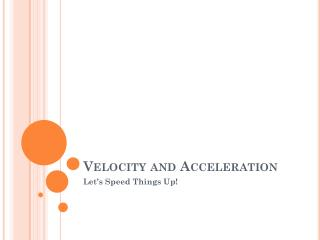Download PresentationVelocity and Acceleration

# Velocity and Acceleration

Download Presentation## Velocity and Acceleration

- - - - - - - - - - - - - - - - - - - - - - - - - - - E N D - - - - - - - - - - - - - - - - - - - - - - - - - - -
##### Presentation Transcript

1. Velocity and Acceleration Let’s Speed Things Up!

2. What is Velocity? • The velocity of an object tells you both its speed and its direction of motion. • A velocity can be positive or negative. • The positive or negative sign for velocity is based on the calculation of a change in position.

3. What is velocity? • Two cars going opposite directions have the same speed, but their velocities are different—one is positive and the other is negative.

4. Velocity Equations • Velocity (v) is calculated by dividing the change in position (Δx) by the change in time (Δt).

5. Example of a Velocity Problem A car moving north in a straight line at constant velocity starts at a position of 10 meters and finishes at 30 meters in five seconds. What is the velocity of the car?

6. What is acceleration? • Acceleration – the rate at which velocity changes • Can be an: • Increase in speed • Decrease in speed • Change in direction

7. Types of acceleration • Increasing speed • Example: Car speeds up at green light • Decreasing speed • Example: Car slows down at stop light • Changing Direction • Example: Car takes turn (can be at constant speed)

8. Formula for acceleration Units for Acceleration = m/s2

9. Acceleration problems 1. A skydiver accelerates from 22 m/s to 45 m/s in 2.0 seconds. What is the skydiver’s average acceleration?

10. Another Example: 2. Calculate the acceleration of a car that slows from 55 m/sec to 38 m/sec in 9.6 seconds?

11. Another example 3. A baby sitter pushing a stroller starts from rest and accelerates at a rate of  0.500m/s2.  What is the velocity of the stroller after it has traveled for 4.75 minutes?